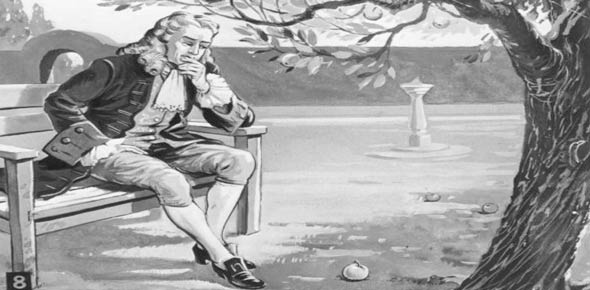# Newton And His Laws

22 Questions | Total Attempts: 434SettingsReviews newton's three laws as well as supporting terms

• 1.
An object at rest wil _______________ if no outside forces are applied.
• A.

At rest

• B.

Increased velocity

• C.

Decreased mass

• 2.
Momentum is related to Newton’s 1st Law
• A.

True

• B.

False

• 3.
More force is required to accelerate an object with a greater mass than a smaller mass.
• A.

True

• B.

False

• 4.
Newton's second law uses the formula...
• A.

E = mc2

• B.

A =pr2

• C.

F = ma

• D.

None of the above

• 5.
Acceleration is the same thing as velocity.
• A.

True

• B.

False

• 6.
Which of Newton's Laws states that an object in motion will stay in motion?
• A.

1st

• B.

2nd

• C.

3rd

• 7.
Gravity always changes the velocity of objects.
• A.

True

• B.

False

• 8.
Which of Newton's Laws is illustrated by a bicyclist riding along, hitting some sand, and flying over the handlebars?
• A.

1st

• B.

2nd

• C.

3rd

• 9.
the acceleration of gravity is ...
• A.

98 m/s2

• B.

9.8 m/s2

• C.

0.9 m/s/s

• 10.
There is always friction when an object is moving on the Earth.
• A.

True

• B.

False

• 11.
Which law explains how rockets are lunched into space?
• A.

Newton's 1st

• B.

Newton's 2nd

• C.

Newton's 3rd

• 12.
A person with a mass of 50 kg would weigh how much on the Earth? (hint F = ma)
• A.

5.1 N

• B.

490 kgm/s

• C.

490 N

• 13.
Air resistance is the fprce air exerts on ...
• A.

Standing objects

• B.

Moving objects

• C.

Objects in space

• 14.
Which of Newton's Laws explain why a person can not throw a softball as far as a baseball.
• A.

1st

• B.

2nd

• C.

3rd

• 15.
A weightlifter raises a 440 kg barbell with an acceleration of 2 m/s2.  How much force does he exert?
• A.

220 N

• B.

5200 N

• C.

968 lbs.

• 16.
You travel from Burlington to Hartford, a distance of 20 miles.  It takes you 40 minutes.  Compute your average speed for the trip.
• A.

20 mph

• B.

30 mph

• C.

50 mph

• 17.
Action-reaction describes which of Newton's three laws?
• A.

1st

• B.

2nd

• C.

3rd

• 18.
Which of the following is a force?
• A.

Inertia

• B.

Friction

• C.

Acceleration

• D.

Velocity

• 19.
What two things determine the amount of gravitational force betwen two objects?
• A.

Mass and distance

• B.

Distance and acceleration

• C.

Mass and inertia

• 20.
The unit for _____________ is m/s2.
• A.

Weight

• B.

Acceleration

• C.

Inertia

• D.

Velocity

• 21.
An object's weight is directly related to its ...
• A.

Volume

• B.

Velocity

• C.

Mass

• D.

Shape

• 22.
A body accelerates if it _____________________.
• A.

Speeds up

• B.

Slows down

• C.

Changes direction

• D.

All of the above

Related TopicsBack to top